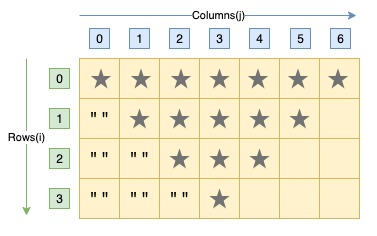# C Program to Print Reverse Pyramid Star Pattern

Here is a C program to print inverted triangle star pattern till n rows using loops. This pattern is also known as inverted pyramid star pattern. For an reversed equilateral triangle pyramid star pattern of 5 rows. Program's output should be:#### Required Knowledge

Algorithm to print inverted pyramid star pattern using loop
This C program is similar to pyramid star pattern, except we are printing the rows in reverse order.
• We first take the number of rows(R) in the pattern as input from user using scanf function.
• Outer for loop(from i = 0 to R-1) will print a row of inverted pyramid in one iteration.
• For jth row, inner for loops first prints i spaces followed by (2*(R-i) - 1) star character.

Here is the matrix representation of the inverted pyramid star pattern. The row numbers are represented by i whereas column numbers are represented by j.## C program to print inverted pyramid star pattern

```#include<stdio.h>

int main() {
int i, j, rows;
printf("Enter the number of rows\n");
scanf("%d", &rows);

for (i = 0; i < rows; i++) {
/* Printing spaces */
for (j = 0; j < i; j++) {
printf(" ");
}
/* Printing stars */
for (j = 0;j < 2*(rows-i)-1; j++) {
printf("*");
}
printf("\n");
}
return 0;
}
```
Output
```Enter the number of rows
5
*********
*******
*****
***
*
```

Related Topics
 C program pyramid star pattern C program hollow pyramid star pattern C program hollow diamond star pattern C program diamond star pattern C program binary rectangle pattern C program natural number triangle pattern C program heart shape star pattern C program hut star pattern C program hollow square star pattern List of all C pattern programs